# FIR Decimation

Filter and downsample input signals

## Library

Filtering / Multirate Filters

`dspmlti4`

•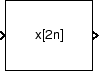## Description

The FIR Decimation block resamples vector or matrix inputs along the first dimension. The FIR decimator (as shown in the schematic) conceptually consists of an anti-aliasing FIR filter followed by a downsampler. To design an FIR anti-aliasing filter, use the `designMultirateFIR` function.

The FIR filter filters the data in each channel of the input using a direct-form FIR filter. The downsampler that follows downsamples each channel of filtered data by discarding M–1 consecutive samples following each sample that is retained. M is the value of the decimation factor that you specify. The resulting discrete-time signal has a sample rate that is 1/M times the original sample rate.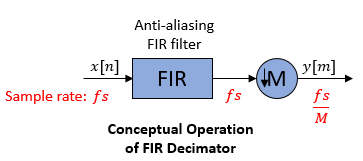Note that the actual block algorithm implements a polyphase structure, an efficient equivalent of the combined system depicted in the diagram. For more details, see Algorithms.

You can use the FIR Decimation block inside triggered subsystems when you set the Rate options parameter to ```Enforce single-rate processing```.

This block supports variable-size input. This means that while the block is simulating, the frame size (number of rows) can change. The output dimensions always equal those of the input signal.

Under specific conditions, this block also supports SIMD code generation. For details, see Code Generation.

### Specifying the Filter Coefficients

To specify the filter coefficients, select the mode you want the FIR Decimation block to operate in. Select the mode in the Coefficient source group box.

• Dialog parameters — Enter information about the filter, such as structure and coefficients, in the block dialog box.

• Input port — Specify the filter coefficients as an input to the block. Coefficient values are tunable (can change during simulation), while their properties must remain constant.

• Filter object — Specify the filter using a `dsp.FIRDecimator` System object™.

• Auto (default) — Choose the filter coefficients of an FIR Nyquist filter, predesigned for the decimation factor specified in the block dialog box.

When you select Dialog parameters, you use the FIR filter coefficients parameter to specify the numerator coefficients of the FIR filter transfer function H(z).

`$H\left(z\right)={b}_{0}+{b}_{1}{z}^{-1}+...+{b}_{N}{z}^{-N}$`

You can generate the FIR filter coefficient vector, b = [b0, b1, …, bN], using one of the DSP System Toolbox™ filter design functions such as `designMultirateFIR`, `firnyquist`, `firhalfband`, `firgr`, or `firceqrip`.

To act as an effective anti-aliasing filter, the coefficients usually correspond to a lowpass filter with a normalized cutoff frequency no greater than 1/M, where M is the decimation factor. To design such a filter, use the `designMultirateFIR` function.

The block internally initializes all filter states to zero.

When you select Auto, the block designs an FIR decimator with the decimation factor specified in Decimation factor. The `designMultirateFIR` function designs the filter and returns the coefficients used by the block.

### Frame-Based Processing

When you set the Input processing parameter to `Columns as channels (frame based)`, the block resamples each column of the input over time. In this mode, the block can perform either single-rate or multirate processing. You can use the Rate options parameter to specify how the block resamples the input:

• When you set the Rate options parameter to `Enforce single-rate processing`, the input and output of the block have the same sample rate. To decimate the output while maintaining the input sample rate, the block resamples the data in each column of the input such that the frame size of the output (Ko) is 1/M times that of the input (Ko = Ki/M),

In this mode, the input frame size, Ki, must be a multiple of the Decimation factor, M.

For an example of single-rate FIR Decimation, see Example 1 — Single-Rate Processing.

• When you set the Rate options parameter to `Allow multirate processing`, the input and output of the FIR Decimation block are the same size, but the sample rate of the output is M times slower than that of the input. In this mode, the block treats a Ki-by-N matrix input as N independent channels. The block decimates each column of the input over time by keeping the frame size constant (Ki=Ko), and making the output frame period (Tfo) M times longer than the input frame period (Tfo = M*Tfi).

See Example 2— Multirate Frame-Based Processing for an example that uses the FIR Decimation block in this mode.

### Sample-Based Processing

When you set the Input processing parameter to `Elements as channels (sample based)`, the block treats a P-by-Q matrix input as P*Q independent channels, and decimates each channel over time. The output sample period (Tso) is M times longer than the input sample period (Tso = M*Tsi), and the input and output sizes are identical.

### Latency

When you use the FIR Decimation block in sample-based processing mode, the block always has zero-tasking latency. Zero-tasking latency means that the block propagates the first filtered input sample (received at time t= 0) as the first output sample. That first output sample is then followed by filtered input samples M+1, 2M+1, and so on.

When you use the FIR Decimation block in frame-based processing mode with a frame size greater than one, the block may exhibit one-frame latency. Cases of one-frame latency can occur when the input frame size is greater than one, and you set the Input processing and Rate options parameters of the FIR Decimation block as follows:

• Input processing = ```Columns as channels (frame based)```

• Rate options = ```Allow multirate processing```

In cases of one-frame latency, you can define the value of the first Ki output rows by setting the Output buffer initial conditions parameter. The default value of the Output buffer initial conditions parameter is `0`. However, you can enter a matrix containing one value for each channel of the input, or a scalar value to be applied to all channels. The first filtered input sample (first filtered row of the input matrix) appears in the output as sample Ki+ 1. That sample is then followed by filtered input samples M+ 1, 2M+ 1, and so on.

Note

### Fixed-Point Data Types

The following diagram shows the data types used within the FIR Decimation block for fixed-point signals.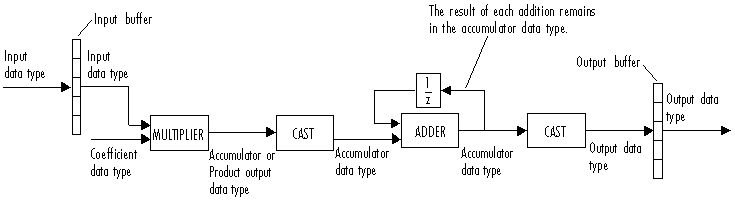You can set the coefficient, product output, accumulator, and output data types in the block dialog box as discussed in the Dialog Box section. This diagram shows that data is stored in the input buffer with the same data type and scaling as the input. The block stores filtered data and any initial conditions in the output buffer using the output data type and scaling that you set in the block dialog box.

When at least one of the inputs to the multiplier is real, the output of the multiplier is in the product output data type. When both inputs to the multiplier are complex, the result of the multiplication is in the accumulator data type. For details on the complex multiplication performed by this block, see Multiplication Data Types.

Note

When the block input is fixed point, all internal data types are signed fixed-point values.

## Examples

### Example 1 — Single-Rate Processing

In the ex_firdecimation_ref2 model, the FIR Decimation block decimates a single-channel input with a frame size of 64. Because the block is doing single-rate processing and the Decimation factor parameter is set to `4`, the output of the FIR Decimation block has a frame size of 16. As shown in the following figure, the input, and output of the FIR Decimation block have the same sample rate.### Example 2— Multirate Frame-Based Processing

In the ex_firdecimation_ref1 model, the FIR Decimation block decimates a single-channel input with a frame period of one second. Because the block is doing multirate frame-based processing and the Decimation factor parameter is set to `4`, the frame period of the output is `4` seconds. As shown in the following figure, the input, and output of the FIR Decimation block have the same frame size, but the sample rate of the output is four times that of the input.### Example 3

The `ex_polyphasedec` model illustrates the underlying polyphase implementations of the FIR Decimation block. Run the model, and view the results on the scope. The output of the FIR Decimation block matches the output of the Polyphase Decimation Filter block.

### Example 4

The `ex_mrf_nlp` model illustrates the use of the FIR Decimation block in several multistage multirate filters.

## Dialog Box

### Coefficient Source

The FIR Decimation block can operate in four different modes. Select the mode in the Coefficient source group box.

• Dialog parameters — Enter information about the filter, such as structure and coefficients, in the block mask.

• Input port — Specify the filter coefficients with a Num input port. The Num input port appears when you select the Input port option. Coefficient values obtained through Num are tunable (can change during simulation), while their properties must remain constant.

• Filter object — Specify the filter using a `dsp.FIRDecimator` System object.

• Auto (default) — Choose the coefficients of an FIR Nyquist filter, predesigned for the decimation factor specified in the block dialog box.

Different items appear on the FIR Decimation block dialog box depending on whether you select Dialog parameters, Input port, Filter object, or Auto in the Coefficient source group box.

## References

 Fliege, N. J. Multirate Digital Signal Processing: Multirate Systems, Filter Banks, Wavelets. West Sussex, England: John Wiley & Sons, 1994.

 Orfanidis, Sophocles J. Introduction to Signal Processing. Upper Saddle River, NJ: Prentice-Hall, 1996.

## Supported Data Types

PortSupported Data Types

Input

• Double-precision floating point

• Single-precision floating point

• Fixed point

• 8-, 16-, and 32-bit signed integers

• 8-, 16-, and 32-bit unsigned integers

Output

• Double-precision floating point

• Single-precision floating point

• Fixed point

• 8-, 16-, and 32-bit signed integers

• 8-, 16-, and 32-bit unsigned integers

expand all

## Algorithms

The FIR decimation filter is implemented efficiently using a polyphase structure. For more details on polyphase filters, see Polyphase Subfilters.

To derive the polyphase structure, start with the transfer function of the FIR filter:

`$H\left(z\right)={b}_{0}+{b}_{1}{z}^{-1}+...+{b}_{N}{z}^{-N}$`

N+1 is the length of the FIR filter.

You can rearrange this equation as follows:

`$H\left(z\right)=\begin{array}{c}\left({b}_{0}+{b}_{M}{z}^{-M}+{b}_{2M}{z}^{-2M}+..+{b}_{N-M+1}{z}^{-\left(N-M+1\right)}\right)+\\ {z}^{-1}\left({b}_{1}+{b}_{M+1}{z}^{-M}+{b}_{2M+1}{z}^{-2M}+..+{b}_{N-M+2}{z}^{-\left(N-M+1\right)}\right)+\\ \begin{array}{c}⋮\\ {z}^{-\left(M-1\right)}\left({b}_{M-1}+{b}_{2M-1}{z}^{-M}+{b}_{3M-1}{z}^{-2M}+..+{b}_{N}{z}^{-\left(N-M+1\right)}\right)\end{array}\end{array}$`

M is the number of polyphase components, and its value equals the decimation factor that you specify.

You can write this equation as:

`$H\left(z\right)={E}_{0}\left({z}^{M}\right)+{z}^{-1}{E}_{1}\left({z}^{M}\right)+...+{z}^{-\left(M-1\right)}{E}_{M-1}\left({z}^{M}\right)$`

E0(zM), E1(zM), ..., EM-1(zM) are the polyphase components of the FIR filter H(z).

Conceptually, the FIR decimation filter contains a lowpass FIR filter followed by a downsampler.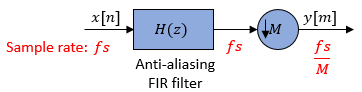Replace H(z) with its polyphase representation.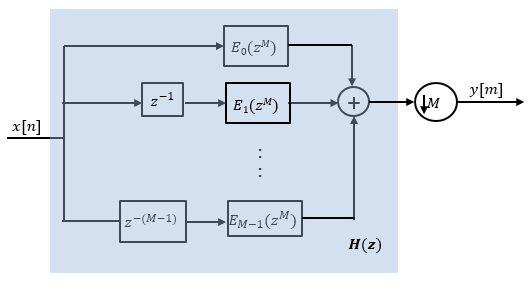Here is the multirate noble identity for decimation.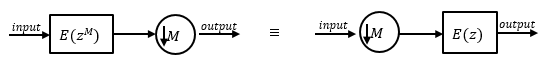Applying the noble identity for decimation moves the downsampling operation to before the filtering operation. This move enables you to filter the signal at a lower rate.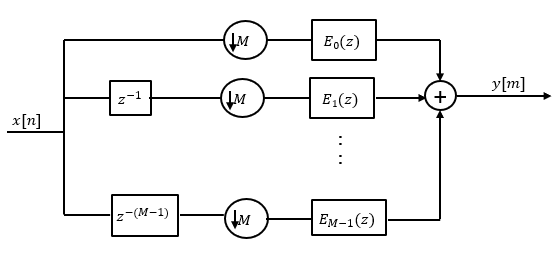You can replace the delays and the decimation factor at the input with a commutator switch. The switch starts on the first branch 0 and moves in the counter clockwise direction as shown in this diagram. The accumulator at the output receives the processed input samples from each branch of the polyphase structure and accumulates these processed samples until the switch goes to branch 0. When the switch goes to branch 0, the accumulator outputs the accumulated value.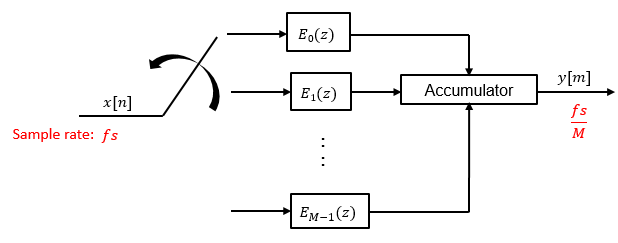When the first input sample is delivered, the switch feeds this input to the branch 0 and the decimator computes the first output value. As more input samples come in, the switch moves in the counter clockwise direction through branches M−1, M−2, and all the way up to branch 0, delivering one sample at a time to each branch. When the switch comes to branch 0, the decimator outputs the next set of output values. This process continues as data keeps coming in. Every time the switch comes to the branch 0, the decimator outputs y[m]. The decimator effectively outputs one sample for every M samples it receives. Hence the sample rate at the output of the FIR decimation filter is fs/M.

## Extended Capabilities

### Fixed-Point ConversionDesign and simulate fixed-point systems using Fixed-Point Designer™.

Introduced before R2006a

## SupportGet trial now